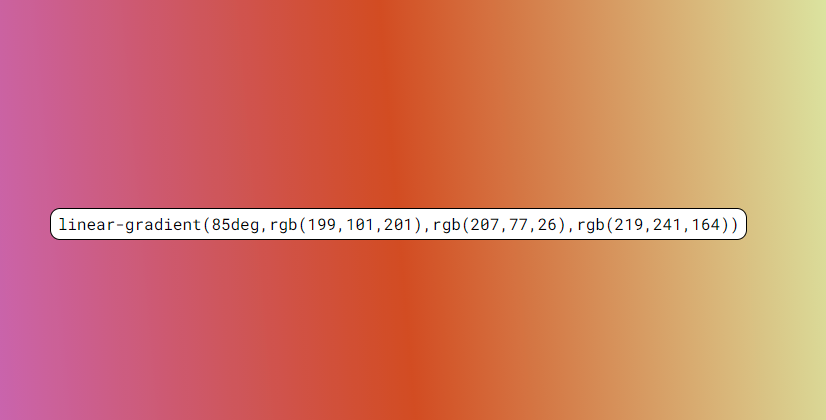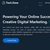## CodeNewbie Community 🌱Jos O'shea (they/them)

Posted on • Updated on

# Coding a random CSS gradient generator!

Over this past weekend I coded a random CSS gradient generator. It was a fun exercise that I relied pretty heavily on the free version of RunJS to get everything working properly. I started with writing a function to generate RGB colors that looked like this:

``````function genRGB() {
const r = Math.floor(Math.random() * 255);
const g = Math.floor(Math.random() * 255);
const b = Math.floor(Math.random() * 255);
return `rgb(\${r},\${g},\${b})`;
};
``````

The code creates a a random RGB color by generating a random number for each of the 3 RGB values assigning them to a variable and then using string template literals to get them in the proper format to be understood as a a RGB value.

After that I wrote a function to use the `genRGB()` function to write a full CSS linear-gradient that could then be applied as a style to the body of the page. That code looked like this:

``````function returnGradient() {
let deg =Math.floor(Math.random() * 360);

};
``````

The first thing `returnGradient()`does is generate an additional random number to be used as the degree angle of the gradient after that I used more string template literals to apply the degree angle that was just generated and then use my previously created function to create the three random colors for the gradient.

the final bit of code that actually applies the CSS gradient as the background of the page looked like this:

``````const h1 = document.querySelector('h1')
});
``````

the first thing this does is select the single `<h1>` tag of the page and assign it to the variable `h1`.
after that I called an event listener to the h1 variable looking for a click. when the h1 is clicked it runs a callback that assigns the `returnGradient()` function to a variable that can be reused. The first thing the variable is used for is to apply the gradient as the `<body>` tag's background1 after that it uses the same gradient variable and changes the h1's text to display the gradient as text on the page. overall it's a pretty simple project but I'm still learning JavaScript and it was a fun learning exercise that looks pretty good from a front-end perspective! you can check out the full project at my Github.

1 applying styles in this manner is not typically considered best practice as it applies them as inline styles which can be unwieldy to work with